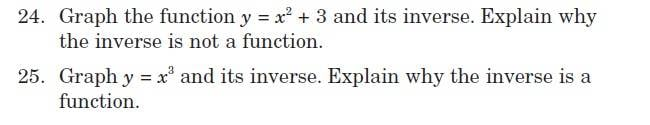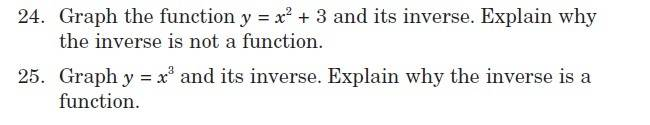# Inverse function of a quadratic and a cubic function?

## Homework Statement## The Attempt at a Solution

for 24. which is a quadratic function, i understand that you have to exchange the positions of x and y and solve from there...but my question is the inverse function of that quadratic for 24 is a negative number under a square root...iv tried google etc... apparently im dealing with imaginary or complex numbers or something... my calculator keeps giving me an error whenever i try to come up with points for the table of values using the inverse function

the inverse function btw is y=±√x-3

how do i get around this? the book clearly shows that you can graph this inverse function but i cant get the y values because the negative number in square root

for 25. the inverse function is y=3√x

using the x values in table of values of -3, -2, -1, 0 ,1 ,2 ,3

can someone please do a few sample calculations and show me how to get the answer? or how they got the solutions for a few of those points? im completely lost for question 25.

thanks

Ray Vickson
Homework Helper
Dearly Missed

## Homework Statement## The Attempt at a Solution

for 24. which is a quadratic function, i understand that you have to exchange the positions of x and y and solve from there...but my question is the inverse function of that quadratic for 24 is a negative number under a square root...iv tried google etc... apparently im dealing with imaginary or complex numbers or something... my calculator keeps giving me an error whenever i try to come up with points for the table of values using the inverse function

the inverse function btw is y=±√x-3

how do i get around this? the book clearly shows that you can graph this inverse function but i cant get the y values because the negative number in square root

for 25. the inverse function is y=3√x

using the x values in table of values of -3, -2, -1, 0 ,1 ,2 ,3

can someone please do a few sample calculations and show me how to get the answer? or how they got the solutions for a few of those points? im completely lost for question 25.

thanks

In 24, you are missing the point completely. Go back to basics: do you know what a function is (an exact definition, please, not some hand-waving statement). Do you know what would be meant by an inverse function (if it existed)? Can you see the trouble in example 24? Hint: it has nothing to do with the fact that for some values of y the equation y = 3 + x^2 has no real solutions; obviously, 3 + x^3 ≥ 3 always, so if we pick a value of y that is < 3 there will be no solution. Again, I emphasize: that is NOT the issue. Go back and answer---very carefully--- the questions I have asked you.

RGV

ok i was able to solve 24 on my own..still no news on 25 though

Mark44
Mentor
Your book should have some discussion about how to determine whether a graph represents a function or not. For example, the graph of y2 = x (or equivalently, y = ±√x) does not represent a function.

yeah, its simple the vertical line test, what i am trying to figure out is how to get the points so i can graph the inverse function for question 25. The book says the inverse function is y=3√x